# C program to add N numbers

In this article, you will learn and get code about how to add n numbers (n integer numbers, n real numbers, and n natural numbers) in C programming. Here, "n" indicates the amount or quantity. That is, how many numbers the user wants to enter and add them as shown here.

For example, if the user enters 5 as the value of n, then the program will further ask the user to enter any 5 number. After receiving 5 numbers from the user, say 10, 20, 30, 40, and 50, the program will sum up all the 5 numbers and display its summation as the result on the output. That will be 150 (because 10 + 20 + 30 + 40 + 50 equals 150).

## Find the sum of n numbers in C

To add n numbers in C programming, you have to ask the user to enter the value of n, then ask to enter n numbers to perform the addition of all the n numbers provided by the user. And then display the addition result as output on the screen. Let's take a look at the program.

```#include<stdio.h>
#include<conio.h>
int main()
{
int i, n, num, sum=0;
printf("Enter the value of n: ");
scanf("%d", &n);
printf("Enter %d numbers: ", n);
for(i=0; i<n; i++)
{
scanf("%d", &num);
sum = sum+num;
}
printf("\nSum of all %d numbers = %d", n, sum);
getch();
return 0;
}```

This program was written in the Code::Blocks IDE. Here is the initial snapshot of the sample run: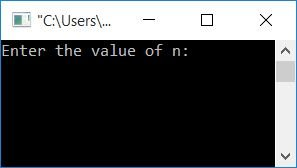Supply the value of n, say 6, then enter any 6 integer values, say 10, 20, 30, 40, 50, or 60, and press the ENTER key to see the output as shown in the second snapshot of the sample run: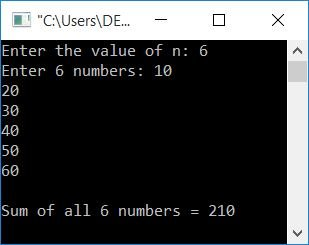#### Steps used in the above program

Some of the main steps used in the above program are:

• Declare the required amount of each variable, that is, i, n, num, and sum.
• Initialize the sum with 0.
• Receive the value of n
• Use the loop (for loop) to receive the desired amount of numbers.
• Inside the for loop, we have applied the summation operation each time when receiving the number.
• That is, when the user enters the first number, say 10, then sum+num or 0+10 gets initialised to sum.
• Again, when the user enters a second number, say 20, then sum+num or 10+20 gets initialised to sum.
• Process the operation for all numbers. That is, if the user enters 6 as the value of n, then the operation goes 6 times.
• Print the value of sum.

### What if the user enters decimal numbers?

The program given above is correct only when the user supplies all the numbers in integer form (without any decimal). So here is the modified version of the above program that is correct for both integer and decimal numbers.

This program is applicable to finding the sum of all real numbers.

```#include<stdio.h>
#include<conio.h>
int main()
{
int n, i;
float num, sum=0;
printf("Enter the value of n: ");
scanf("%d", &n);
printf("Enter %d numbers: ", n);
for(i=0; i<n; i++)
{
scanf("%f", &num);
sum = sum+num;
}
printf("\nSum of all %d numbers = %0.2f", n, sum);
getch();
return 0;
}```

Here is a snapshot of the first sample run. In this sample run, we have provided all the numbers as an integer value: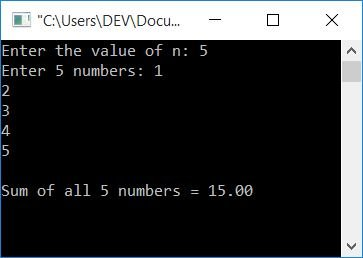And here is the snapshot of the second sample run. In this sample run, we have provided all the numbers as floating-point values: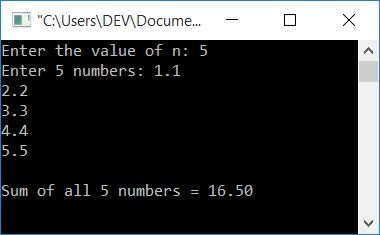In the above program, we only made these changes:

• Declared the num and sum variables as float data types for real number operations.
• For both variables, %f format specifier used here
• 0.2f is used to print the value of sum upto 2 decimal places
• Rest of the program is the same as the above one

## C program to find the sum of N natural numbers

As we all know, positive numbers 1, 2, 3,... are natural numbers. Therefore, here we only have to ask the user for the value of n, that is, up to how many terms the natural number continues, and find the sum of that natural number.

For example, if the user enters the value of n as 8, then find the sum of the first eight natural numbers, which will be 1, 2, 3, 4, 5, 6, and 8. Let's take a look at the program:

```#include<stdio.h>
#include<conio.h>
int main()
{
int i, n, sum=0;
printf("Enter the value of n: ");
scanf("%d", &n);
for(i=1; i<=n; i++)
sum = sum+i;
printf("\nSum of %d natural numbers = %d", n, sum);
getch();
return 0;
}```

Here is a snapshot of the sample run: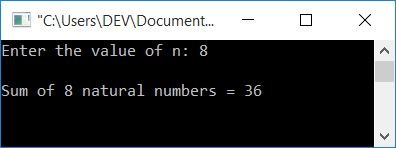#### The same program in different languages

C Quiz

« Previous Program Next Program »